## Resource of the Week: Maths SAT questions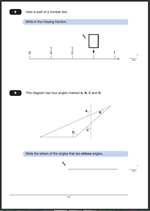For anyone in Year 6 the Maths SAT tests will be coming up before too long! Don’t forget that we have a whole section of past questions and answers, including great explanations of the best ways to approach each question. Invaluable help to achieve those higher levels!

This week I am highlighting are two questions from the Maths SAT Paper 2011 Paper B. The first involves equivalent fractions and the second angles and shape.

The answer to Question 8.   3/4
One mark awarded for a correct answer.
Equivalent fractions accepted as is 0.75.
Suggested method:
This question is another reading a number line problem and if children can count in quarters it is an easy mark. Firstly, it has to be recognised that the number line is showing quarters (1/2 is equivalent to 2/4 and 1 is equivalent to 4/4) making the missing number 3/4.

The answer to Question 9. A and D.
One mark awarded for a correct answer.
The letters can be given in either order. Both need to be given with no incorrect angles added.
Suggested method:
Answering this question correctly depends on 3 things:
Firstly, a recognition of the conventions for labelling angles, using the arc.
Secondly, a knowledge that an obtuse angle is larger than 90 degrees but less than 180 degrees.
If either of these are unknown it becomes purely a guessing game. (Some children do think that the angle depends on the length of the lines rather than a measure of turn.)

Thirdly, if these are known, then the obtuse angles still need to be recognised. One way to do this is to slide a right angle (eg a corner of a piece of paper) into the angle to see whether it is smaller or larger.

Why not visit ks2-maths-sats.co.uk for free SATs papers and a great SAT revision programme?

Questions 8 and 9 from SAT Paper B 2011

Questions 8 and 9 answers and suggested method 2011 Paper B

## Maths SAT Paper 2011 Paper B Questions 8 and 9Here are two more questions from the Maths SAT Paper 2011 Paper B.

The answer to Question 8.   3/4
One mark awarded for a correct answer.
Equivalent fractions accepted as is 0.75.
Suggested method:
This question is another reading a number line problem and if children can count in quarters it is an easy mark. Firstly, it has to be recognised that the number line is showing quarters (1/2 is equivalent to 2/4 and 1 is equivalent to 4/4) making the missing number 3/4.

The answer to Question 9. A and D.
One mark awarded for a correct answer.
The letters can be given in either order. Both need to be given with no incorrect angles added.
Suggested method:
Answering this question correctly depends on 3 things:
Firstly, a recognition of the conventions for labelling angles, using the arc.
Secondly, a knowledge that an obtuse angle is larger than 90? but less than 180?.
If either of these are unknown it becomes purely a guessing game. (Some children do think that the angle depends on the length of the lines rather than a measure of turn.)

Thirdly, if these are known, then the obtuse angles still need to be recognised. One way to do this is to slide a right angle (eg a corner of a piece of paper) into the angle to see whether it is smaller or larger.

Why not visit ks2-maths-sats.co.uk for free SATs papers and a great SAT revision programme?

Questions 8 and 9 from SAT Paper B 2011

Questions 8 and 9 answers and suggested method 2011 Paper B

## KS2 Maths SAT Paper B question 6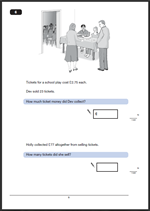Question 6 of the KS2 maths SAT Paper B is in two parts and it would be most sensible to use a calculator.

The first part of the question looks at the cost of 23 tickets at £2.75 each.

The answer to question 6a is  £63.25
One mark awarded for a correct answer.
Any unambiguous indication of the correct amount is acceptable, including: £63.25p, £63.25 pence or £63,25.

Suggested method:

This question is mainly a matter of deciding what process to carry out and using a calculator accurately.
Some children have very little practice with a calculator and may be tempted not to use it.
Recognising that this is a multiplication question and carefully keying in the correct amount, including the decimal point should ensure a correct answer is reached.
Remind children it is always worth carrying out a reverse operation to check that the answer is correct. In this case £63.25 divided by 23 will give £2.75.

The second part gives the total amount from ticket sales and asks how many tickets were sold.

The answer to question 6b is 28.
One mark awarded for a correct answer.

Suggested method:

Easy one mark use a calculator to divide £77 by 2.75. Perhaps the one major error that children can make is to put the numbers in the wrong way round on the calculator (2.77 ÷ 77).

Why not visit ks2-maths-sats.co.uk for free SATs papers and a great SAT revision programme?

Question 6

Question 6 answers and suggested method 2011 paper B

## KS2 Maths 2011 Paper B: Question 5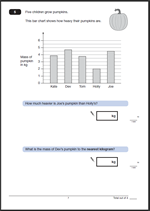Here we have question 5 from the KS2 maths 2011 Paper B. There are two parts to the question, both based on interpreting a block graph.

The answer to 5a is:   2.5 or 2 1/2

One mark awarded for a correct answer.

Suggested method:

This is a straightforward graph question which should prove to be an easy mark to gain.

The important part of this question is to look carefully at the scale showing the mass of the pumpkin in kilos. Every other line is marked in kilos, so every other line must be a half kilo.

Joe’s pumpkin has a mass of 4.5 kg. Holly’s has a mass of 2 kg. The difference is 4.5 kg – 2 kg = 2.5 kg.

Note the use of mass rather than weight in this question. Children should be very familiar with this.

The answer to 5b is: 5 kg

One mark awarded for a correct answer.

Suggested method:

This is a rounding question. The first thing to do is to look at the mass of Dev’s pumpkin. It is between 4.5 kg and 5 kg. As it is more than 4.5 kilos the mass should be rounded up to 5 kg (to the nearest whole kilogram).

Question 5 from Paper B 2011

Question 5 answers and suggested method 2011 Paper B

Why not visit ks2-maths-sats.co.uk for free SATs papers and a great SAT revision programme?

## KS2 maths 2011 paper B question 4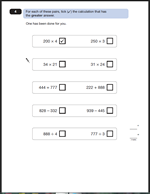We continue our look at the KS2 Maths 2011 Paper B with this question, which should be an easy 2 marks, but all too often little errors are made when using a calculator.

Two marks awarded for all four rows correct.

One mark awarded for 3 out of 4.

No marks for 2 or less correct.

Other clear indications of the answer, such as a cross or Y will also gain the mark.

Suggested method:

The key to answering this question is to remember that a calculator is available and to take each pair of questions separately.

Children should be able to use the keys properly. They should know how to clear the calculator, how to enter numbers and operations and how to read the results. Interpreting the results is also important and it is essential to look at the answer to check that it is sensible – within a range of what is expected.

For example 34 x 21 is going to be around 600 or 700.

With these questions it is a good idea to jot down each of the two answers and then compare them. It is essential that the calculator is cleared after each calculation.

Question 4 from SAT Paper B 2011

Question 4 answers and suggested method 2011 paper B

## KS2 Maths 2011 Paper B: Question 3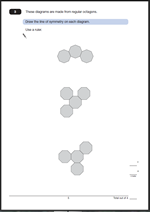Symmetry is a very popular subject with the SAT writers and can be an easy way to pick up a couple of marks.

For this question two marks are awarded for all three lines of symmetry drawn correctly.
One mark awarded for two diagrams completed correctly.
No marks for just one marked correctly.
Slight inaccuracies in drawing can be allowed, provided the intention is clear. A ruler should be used.
Suggested method:
This is very much a case of using the resources that you have been provided with and recognising how they can be useful.

A mirror is certainly helpful for this question. Placing it where it is thought the line of symmetry should be and looking to see if the reflection matches the drawing. When working out where the lines should go imagine that they are folded along the line and completely overlap each other. Some children will find this very tricky, especially as two of the lines of symmetry are not horizontal or vertical.
Alternatively, use tracing paper to draw the diagrams and then fold so that the two sides completely overlap. Draw the line where the fold is. Tracing paper is often provided in the test.

Question 3

Question 3 answer and suggested method 2011 paper B

## KS2 Maths SAT questions and answers: Paper B 2011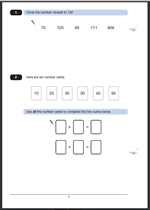This is the first in a new series looking at SAT papers, the questions, answers and best methods of going about them. This term I am looking at the 2011 Paper B. Calculators are allowed for this paper, but generally I think that paper B tends to be harder than Paper A.

The first question has 5 numbers and asks which is the nearest to 100.

1.   The number 111 should be circled.
One mark for this question.
Other clear indications of the answer, such as underlining or ticking will also gain the mark.
Suggested method:
This is probably best done by a process of elimination.

909 can immediately be crossed off as it is so far from 100.

This leaves 70 and 85 below 100
and 111 and 120 above 100.

Immediately it can be seen that 85 is nearer to 100 than 70 and that 111 is nearer than 120.
So that leaves 85 and 111.

85 is 15 away from 100. 111 is 11 away from 100 so the answer must be 111.

2. 10 + 30 = 40

20 + 30 = 50

One mark for this question.
Calculations may be given in either order.

The first two numbers within each calculation may be given in either order.
Suggested method:
A quick glance at the numbers on the cards will suggest that the answer cards are likely to have the two highest numbers in them. That’s 40 and 50.
50 can be made from 30 and 20. This leaves 30 and 10 to make 40.

A calculator is not really of any help with these two questions.

2011 Paper B Question 1 and 2

Question 1 and 2 answers and suggested method 2011 Paper B

## KS2 Maths SAT question 25 from 2010 Paper A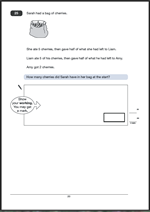Sarah had a bag of cherries…..Question 25 is one of those questions where you have to read it all carefully before starting and then proceed from the end, working backwards.

Suggested method:

The key to answering this question is to start at the end and work backwards.
Amy got 2 cherries.
Liam gave half of what he had left to Amy, so Liam must have had 4 left.
Liam had eaten 5 of his cherries so he must have had 5 + 4 = 9 cherries.
Sarah gave half of what she had left to Liam. This was 9 so she must have had 18 left.

Two marks for this question.

If the answer is incorrect one mark can be given for showing appropriate working out, such as
2 x 2 = 4          4 + 5 = 9          9 x 2 = wrong answer of 16              16 + 5 = wrong answer of 21.
The working out must be carried through to reach an answer.

This is very much at the level 5 end of the paper, so correct marks here could just make the difference between Level 4 and level 5.

Question 25 from SATs Paper A 2010

Question 25 answer and suggested method

## KS2 Maths SAT questions 23 and 24 from 2010 Paper A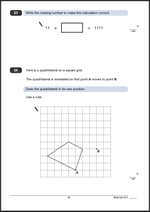To answer question 23 you need to find the missing number to make the calculation correct.

11 x ?? = 1111

One mark for this question.

Suggested method:
To make this easy a good understanding of the relationship between multiplication and division is needed. Simply divide 1111 by 11, which is probably best done using the short division method.

It is possible that some children will recognise the pattern of dividing by 11 and immediately know this answer.

Question 24 asks for a quadrilateral to be drawn in its new position after a translation.

One mark for this question.

Slight inaccuracies in drawing are allowed (within 2 mm radius of correct points).

Suggested method:

The key to this question is the word ‘translated’. A translation is a slide in a particular direction. There is no rotation or change in the shape size.

This shape has been slid 3 squares to the right and 3 squares up.

Using this knowledge mark each of the other three points and join them with a ruler.

Questions 23 and 24 from SATs Paper A 2010

Questions 23 and 24 answers and suggested methods

Why not visit ks2-maths-sats.co.uk for free SATs papers and a great SAT revision programme?

## KS2 Maths SAT question 22 from 2010 Paper AQuestion 22a and 22b look at interpreting pie charts.

For 22a  An acceptable answer is within the range of 13/100 to 1/5 inclusive.
This range includes the fractions 1/6 and 1/7.
Decimals can be accepted within the range 0.13 to 0.2 inclusive.
Percentages can be accepted within the range 13% to 20% inclusive.
One mark for this question.
Suggested method:
This can be thought of as a visual question. Split the circle in half by continuing the vertical line down (between Books and DVDs.).  From this it can be seen that DVDs are just about 1/3 of the half, so will be about 1/6 of the whole circle. Because it is only an estimate there is a fair range to be within.

For 22b Answers in the range of 500 to 800 inclusive.
One mark for this question.

Suggested method:
Again this can be done by comparing the size of the two. Book sales are about 3 times that of CDs. So if 200 CDs were sold then about 600 books were sold.

Question 22 from Maths Paper A 2010

Question 22 answers and suggested methods.